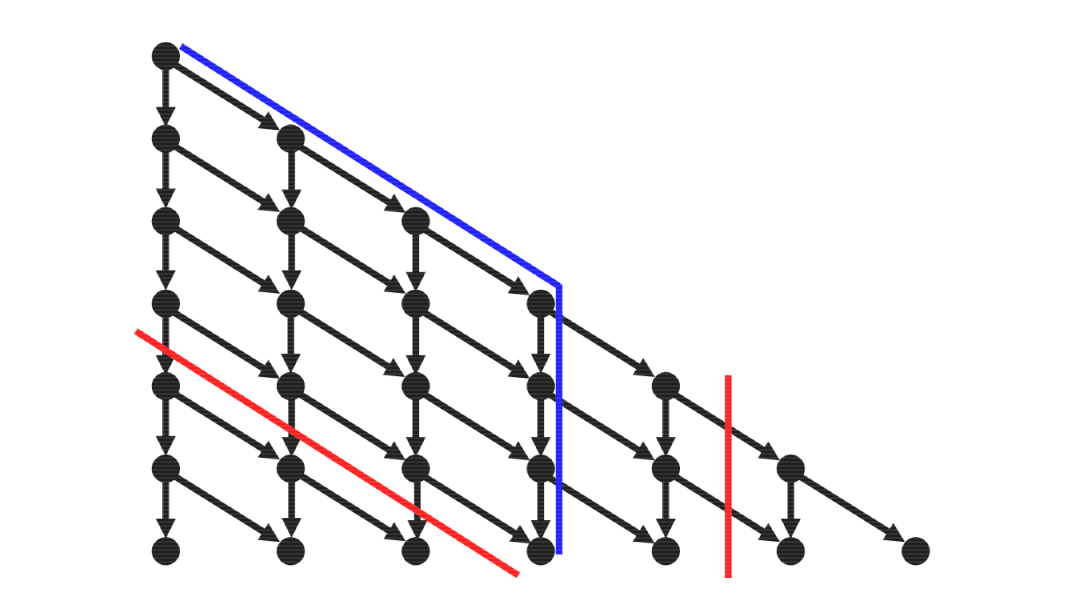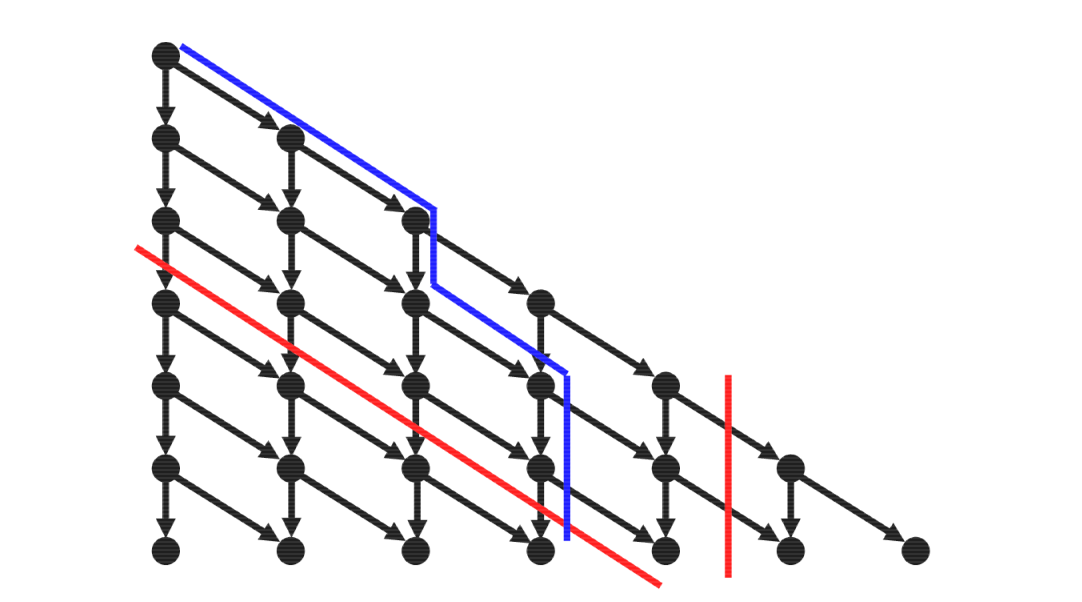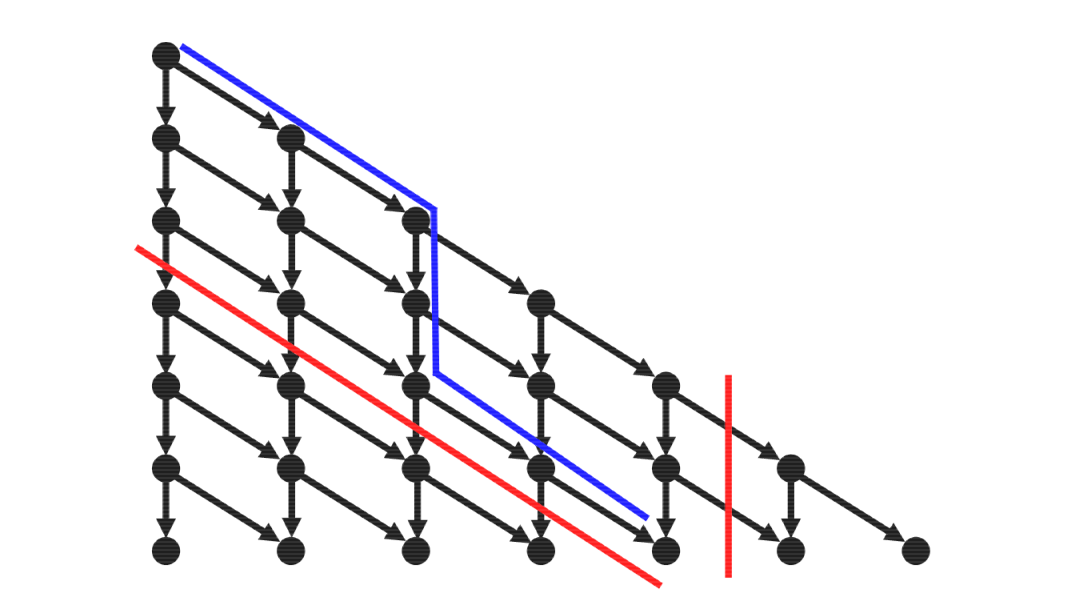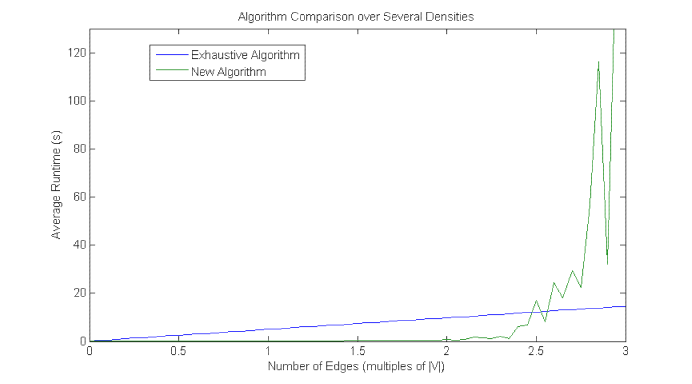# A combinatorial algorithm for finding maximum cuts  (Page 3/3)

 Page 3 / 3

Several researchers have published algorithms that attempt to improve performance, especially focusing on sparse graphs. Notable approaches include those of Wheeler with ${O}^{*}\left({2}^{|V|}\right)$ [link] , Fedin and Kulikov with ${O}^{*}\left({2}^{|E|/4}\right)$ [link] , Croce, Kaminski, and Paschos with ${O}^{*}\left({2}^{|V||E|/|V|+|E|}\right)$ , and Williams with ${O}^{*}\left({2}^{\omega |V|/3}\right)$ , $\omega <2.376$ [link] . Although the last algorithm has the best computational complexity, it requires exponential space while the others require only polynomial bounded space. Due to limited memory storage capacity, algorithms requiring only polynomial bounded space are highly preferable.

## A new algorithm

In attempt to improve performance for sparse graphs, this research presents a new exact algorithm for finding maximum cuts of unweighted graphs that will now be described. It requires polynomial bounded space and has computational complexity $O\left(|E|{2}^{|E|}\right)$ . The general approach that this algorithm takes is to separate the graph into its connected components and calculate initial upper and lower bounds for the maximum cut of each connected component. Those initial bounds are then used with a branch and bound algorithm to find a maximum cut of each connected component, and the maximum cuts of the connected components are be combined to find the maximum cut of the original graph.

In order to accomplish this, let I be the set of edge induced subgraphs of graph $G\left(V,E\right)$ . Let E be indexed by $\mathbb{Z}\left[1,|E|\right]$ according to some ordering. Let I be indexed by $\mathbb{Z}\left[0,{2}^{|E|}-1\right]$ according to the bijective function $f:\mathbb{Z}\left[0,{2}^{|E|}-1\right]\to I$ such that

${f}^{-1}\left({G}_{1}\left({V}_{1},{E}_{1}\right)\right)=\sum _{i=1}^{|E|}\left\{\begin{array}{cc}0& {e}_{i}\in {E}_{1}\hfill \\ {2}^{|E|-i}& {e}_{i}\notin {E}_{1}\hfill \end{array}\right)$

This indexing provides the advantage that the size of each element is the number of zeros in the binary representation of the index. Thus, it enables the use of the edge and graph indices to rapidly eliminate subgraphs that cannot be bipartite and surpass the current lower bound for the maximum cut.

Within this framework, the algorithm searches for the lowest indexed element of I that is bipartite and larger than the current lower bound by iterating over the edges in order, adding each edge to the cut set if it passes a parity check and stopping when the final edge is reached or the number of edges not cut ensures the result will not exceed the lower bound. If such an element is found, the size of the graph is a new lower bound for the maximum cut, and the search continues by removing last cut edge with at least one vertex not colored by a lesser indexed edge before last two edges not in cut set. Continue iterating from that edge if such an edge is found, but terminate if no such edge exists or a cut equal to the upper bound is reached. Otherwise, the search ends and the final lower bound is the size of the maximum cut.

This process can be visualized as a directed graph, like those in [link] , [link] , and [link] , where each step represents a decision whether to include an edge in the cut set. A movement directly downward from a node indicates that the corresponding edge is not cut, while a movement diagonally downward indicates that the corresponding edge is cut. Each path from the top node to one of the bottom nodes corresponds to an edge induced subgraph of the graph being examined. The number of the final node reached (from left to right starting with 0) is the number of edges included. The algorithm seeks to find a path corresponding to a bipartite subgraph that leads farthest to the right. The red line to the left represents the lower bound, and all nodes below this line cannot be part of a path that leads farther right than the lower bound. The red line to the right represents the upper bound, and all nodes to the right of this line can only be a part of paths that exceed the upper bound. Therefore these regions need not be explored. An illustrative example, corresponding to an instance of K 4 with an edge ordering conductive to demonstration where a lower bound of 2 and an upper bound of 4 have been initially calculated (poor lower bound chosen for purpose of demonstration), is shown in [link] , [link] , and [link] .This image illustrates the process for a K 4 (with poorly estimated lower bound for purposes of demonstration). Each step represents a decision on including the corresponding edge. Each path from top to bottom, like that in blue, corresponds to an edge induced subgraph. The red lines correspond to upper and lower bounds.The algorithm searches for paths that lead further to the right than previously found. There is no need to search in regions beyond the red lines indicating bounds, which have been updated from the initial lower bound after finding a larger solution in the previous figure.Finally, a solution leading further right is found. Because this solution is of equal size to the upper bound, the search ends since there cannot be a larger cut.

## Empirical testing

This new algorithm was compared to the exhaustive algorithm for performance in empirical testing as shown in [link] . The image shows a plot of average runtime for graphs with $|V|=20$ nodes and numbers of edges between 0 and $3|V|=60$ . Each data point for each algorithm is the average of runtimes for five randomly generated graphs with the same number of vertices. In the testing, the new algorithm outperformed the exhaustive algorithm at low densities about until $|E|<2.5|V|$ , successfully improving performance over the exhaustive algorithm at sufficiently low densities. However, no comparison to other algorithms developed by other researchers has been performed, and the algorithm is not expected to match or improve upon their performance.This image shows a plot of average runtime for graphs with | V | = 20 nodes and numbers of edges between 0 and 3 | V | = 60 . Each data point for each algorithm is the average of runtimes for five randomly generated graphs with the same number of vertices.

## Conclusion

Finding the maximum cut of a graph is a difficult to compute problem in combinatorial optimization with several applications in the world of engineering and physics. This research develops and evaluates an exact branch and bound algorithm for the maximum cut of unweighted graphs that was designed for improved performance on sparse graphs. Although the algorithm developed provides a performance improvement over the exhaustive algorithm, it is not expected to perform as well or better than other algorithms developed by other researchers. Thus, further improvement of the algorithm, focusing on investigating the effect of edge orderings on the performance of the algorithm and finding additional measures to reduce the number of edge induced subgraphs traversed by the algorithm, and more extensive empirical evaluation are necessary.

where we get a research paper on Nano chemistry....?
nanopartical of organic/inorganic / physical chemistry , pdf / thesis / review
Ali
what are the products of Nano chemistry?
There are lots of products of nano chemistry... Like nano coatings.....carbon fiber.. And lots of others..
learn
Even nanotechnology is pretty much all about chemistry... Its the chemistry on quantum or atomic level
learn
da
no nanotechnology is also a part of physics and maths it requires angle formulas and some pressure regarding concepts
Bhagvanji
hey
Giriraj
Preparation and Applications of Nanomaterial for Drug Delivery
revolt
da
Application of nanotechnology in medicine
what is variations in raman spectra for nanomaterials
ya I also want to know the raman spectra
Bhagvanji
I only see partial conversation and what's the question here!
what about nanotechnology for water purification
please someone correct me if I'm wrong but I think one can use nanoparticles, specially silver nanoparticles for water treatment.
Damian
yes that's correct
Professor
I think
Professor
Nasa has use it in the 60's, copper as water purification in the moon travel.
Alexandre
nanocopper obvius
Alexandre
what is the stm
is there industrial application of fullrenes. What is the method to prepare fullrene on large scale.?
Rafiq
industrial application...? mmm I think on the medical side as drug carrier, but you should go deeper on your research, I may be wrong
Damian
How we are making nano material?
what is a peer
What is meant by 'nano scale'?
What is STMs full form?
LITNING
scanning tunneling microscope
Sahil
how nano science is used for hydrophobicity
Santosh
Do u think that Graphene and Fullrene fiber can be used to make Air Plane body structure the lightest and strongest. Rafiq
Rafiq
what is differents between GO and RGO?
Mahi
what is simplest way to understand the applications of nano robots used to detect the cancer affected cell of human body.? How this robot is carried to required site of body cell.? what will be the carrier material and how can be detected that correct delivery of drug is done Rafiq
Rafiq
if virus is killing to make ARTIFICIAL DNA OF GRAPHENE FOR KILLED THE VIRUS .THIS IS OUR ASSUMPTION
Anam
analytical skills graphene is prepared to kill any type viruses .
Anam
Any one who tell me about Preparation and application of Nanomaterial for drug Delivery
Hafiz
what is Nano technology ?
write examples of Nano molecule?
Bob
The nanotechnology is as new science, to scale nanometric
brayan
nanotechnology is the study, desing, synthesis, manipulation and application of materials and functional systems through control of matter at nanoscale
Damian
Is there any normative that regulates the use of silver nanoparticles?
what king of growth are you checking .?
Renato
What fields keep nano created devices from performing or assimulating ? Magnetic fields ? Are do they assimilate ?
why we need to study biomolecules, molecular biology in nanotechnology?
?
Kyle
yes I'm doing my masters in nanotechnology, we are being studying all these domains as well..
why?
what school?
Kyle
biomolecules are e building blocks of every organics and inorganic materials.
Joe
how did you get the value of 2000N.What calculations are needed to arrive at it
Privacy Information Security Software Version 1.1a
Good
Got questions? Join the online conversation and get instant answers!By Brooke DelaneyBy OpenStaxBy OpenStaxBy Stephen VoronBy OpenStaxBy Madison ChristianBy Dakota BocanBy OpenStaxBy Rohini AjayBy Stephen Voron